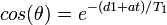# Ernst angle

The Ernst angle is the tip angle for a particular spin that will yield the maximum signal in the least amount of time when signal averaging over many transients. The the following equation relates the Ernst angle, theta, to the experimental interpulse delay, d1; the duration of the fid acquisition, at; and the T1 relaxation time of the spin in question, T1:$cos(\theta)=e^{-(d1+at)/T_1}$

This relationship was developed by Richard Ernst, winner of the 1991 Nobel Prize in Chemistry.

For example, if one wishes to get the best signal from a resonance with T1=3 sec, and one wishes to use d1=1sec and at=2sec, the optimal tip angle is 68 degrees.

### References

1. Ernst, RR and Anderson, WA. Application of Fourier transform spectroscopy to magnetic resonance. Review of Scientific Instruments 37:93, 1966. BibTeX [ernst1]